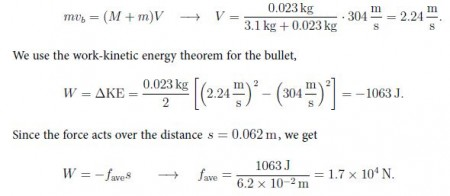# Define Collisions with Mathematical Exampls

The momentum is conserved, but the mechanical energy is not conserved in the example with a person throwing the dumbbell while standing on a skateboard. This type of interaction between objects is called inelastic collisions.

Example: A bullet [with mass m = 23 g and velocity vb = 304 m/s] is fired into a block [with mass M = 3.1 kg] that is initially at rest. The bullet embeds itself 6.2 cm into the block a) What is the speed of the block after the bullet embeds itself? b) What is the average force exerted by the block on the bullet?

Solution: This is an inelastic collision, so that for the speed V,When both momentum and mechanical energy are conserved, the collisions are said to be completely elastic,

M1 v1i + m2 v2i = M1 v1f + m2 v2f

(m1/2) v21i + (m2/2) v22i = (m1/2) v21f + (m2/2) v22f

We thus have two equations for two unknowns v1f and v2f, assuming that the masses of the two colliding objects are known, (v1i, v2i) → (v1f, v2f).